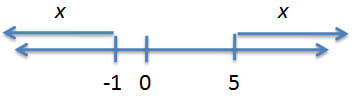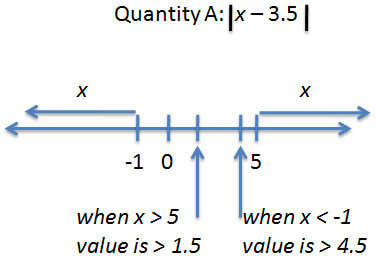Manipulating Inequalities and Absolute Value on the GRE

Did you know that you can attend the first session of any of our online or in-person GMAT courses absolutely free? We’re not kidding! Check out our upcoming courses here.

Most people dislike absolute value, and inequalities can tie us up into knots. Put them together, and we can have some major headaches! Let’s test one out.

Set your timer for 1 minute and 15 seconds for this Quantitative Comparison problem and GO!

* |x — 2| > 3

Quantity A                                                                 Quantity B

The minimum possible                                              The minimum possible

value of |x — 3.5|                                                        |x — 1.5|

What did you get? (Do you remember the 4 QC answer choices? I didn’t list them above! If you don’t know what they are, go look them up. I’ll wait. And the pain of having to look them up will help convince you that you need to memorize these.)

We have a given:

|x — 2| > 3

So, first, let’s figure out what this actually means. For what values of x is this inequality true?

When an inequality or an equation contains an absolute value sign, we have to think of this as two equations (or inequalities). The first one is the actual inequality that we were given, without the absolute value sign:

x — 2 > 3

The second one is the negative of the first one. Choose one side (it doesn’t matter which one, but it’s easiest to choose whichever side is simpler) and make it negative. If you have an equation (= sign), then that’s all you need to do. If you have an inequality, though, then things are a bit more complicated. With inequalities, we also have to reverse the direction of the inequality (think of it as multiplying by a negative). So, in the above case, we would get this:

x — 2 < -(3)

You’ll notice that I put parentheses around the 3. I don’t really need to do that in this case, because it’s only a 3, but this could make a difference on a different problem, so it’s a good idea to get into the habit of including parentheses, just in case.

All right, we have these two equations:

x — 2 > 3

x — 2 < -(3)

Simplify each one. x — 2 > 3 becomes x > 5. And x — 2 < -(3) becomes x < -1. The original equation, then, is telling us that x could be greater than 5 or less than -1.

Because we’re dealing with absolute value in general, it might be useful to illustrate this on a number line (particularly because, if we glance at Quantities A and B, we can see that we’re not done with absolute value yet!). Our number line will include 0 not only because we always include 0 on number lines but also because the question is about absolute value—which means negative vs. non-negative is a key issue here.What does absolute value mean again? Absolute value turns negative numbers positive (or, in the case of 0, leaves the number the same). Absolute value is really telling us the distance a number is from zero on the number line, regardless of direction. What’s the closest possibility for x? On the -1 side, x could be just a bit more than 1 unit away from zero.

Take any number in the world and put an absolute value symbol around it. What’s the smallest possible value you can think of, once the absolute value symbol has been applied?

Right. Zero. The absolute value of zero is zero. The absolute value of anything else is at least a tiny bit bigger than zero, because absolute value gets rid of any negative signs. So the smallest possible value for anything inside an absolute value symbol is zero.

Now, why did I ask you that? Take a look at Quantity A:

The minimum possible value of |x — 3.5|

I asked you that because that’s what the problem wants me to find: the minimum possible value once that absolute value sign has been applied. Can we make it come out to zero? What would x have to be in order for the overall value to be zero?

The value of x would have to be 3.5. Now, I know I can’t make x = 3.5 because, glancing at my number line, I can see that x has to be bigger than 5 or smaller than -1. Of the possible values for x, which is closest to 3.5?

We should look at the line that start at a little bit bigger than 5. What if we plug in this value?

|slightly bigger than 5 — 3.5|

Do the math but keep the “slightly bigger than” language: that would equal something |slightly bigger than 1.5| or > 1.5. The value in Quantity A, then, is something just slightly bigger than 1.5.

What about Quantity B? Use the same thought process. In order for the value of |x — 1.5| to be zero, x would have to be 1.5. It can’t be 1.5, but what’s the closest possible value that it can be? In this case, we need to go in the other direction: 1.5 is closer to -1 than it is to +5.

This time, we’re doing this math:

|slightly smaller than -1 — 1.5|

|slightly smaller than -2.5|

Now here’s a weird little twist: I know I’m going to drop the negative sign since I’ve got an absolute value symbol, right? Think of this as dividing by a negative: we also need to flip the inequality sign. So this becomes: slightly larger than +2.5 or > 2.5.

Which is the larger value? Quantity B. The correct answer is B.

Now, you might look at all of that and think, I can’t think it through like that. I’d mess that up. If so, that’s okay. Here’s another (slightly longer) way to approach it. You’ll have to test more cases, but you might find the process more straightforward.

Do everything the same up to the point where we began examining Quantity A. We know that x > 5 and x < -1, and we've drawn our number line. Then test both ends of the possible ranges (slightly less than -1 and slightly more than 5) for both Quantity A and Quantity B.

First, try the -1 end of the range. x < |-1 — 3.5| < |-4.5|. Next, apply the absolute value symbol. If x < |-4.5|, then applying the absolute value symbol gives us x > +4.5 (remember, we flip both the sign and the inequality symbol). Next, try 5: x > |5 — 3.5| > |1.5|. We don’t need to flip the sign this time because the number is already positive. x > 1.5. Here’s how it would look on the number line.So for Quantity A, the smallest possible value (the one closest to zero) is something just a bit bigger than 1.5. Now, do the same thing for Quantity B: test both ends of the range.

This time, we’ve got x < |-1 — 1.5| < |-2.5|. Applying the absolute value symbol to this negative value, we get x > 2.5. The other possibility is x > |5 — 1.5| > |3.5|, or x > 3.5. In this case, the smallest possible value (the one closest to zero) is something just a bit bigger than 2.5, so Quantity B is greater and the answer is B.

Key Takeaways for Inequality and Absolute Value Problems

(1) Equations or inequalities containing absolute value symbols actually represent two different equations (or inequalities), not just one. Make sure that you’re solving for both!

(2) If you have an inequality inside of an absolute value symbol (as we did when we tested possible values here), you have to flip the sign when the value of the number is negative—just as we would if we were solving a normal inequality.

(3) Try drawing things out. Absolute value problems are really about negative vs. non-negative, so a number line will often help to sort out what the problem is really telling/really asking. ?

Can’t get enough of Stacey’s GMAT mastery? Attend the first session of one of her upcoming GMAT courses absolutely free, no strings attached. Seriously.Stacey Koprince is a Manhattan Prep instructor based in Montreal, Canada and Los Angeles, California. Stacey has been teaching the GMAT, GRE, and LSAT  for more than 15 years and is one of the most well-known instructors in the industry. Stacey loves to teach and is absolutely fascinated by standardized tests. Check out Stacey’s upcoming GMAT courses here.

1.prat k October 4, 2015 at 11:03 pm

your article is full of double quote marks which makes it unreadable

2.www.gimnazijatg.com March 30, 2013 at 6:41 am

yktoiNCq Phentermine